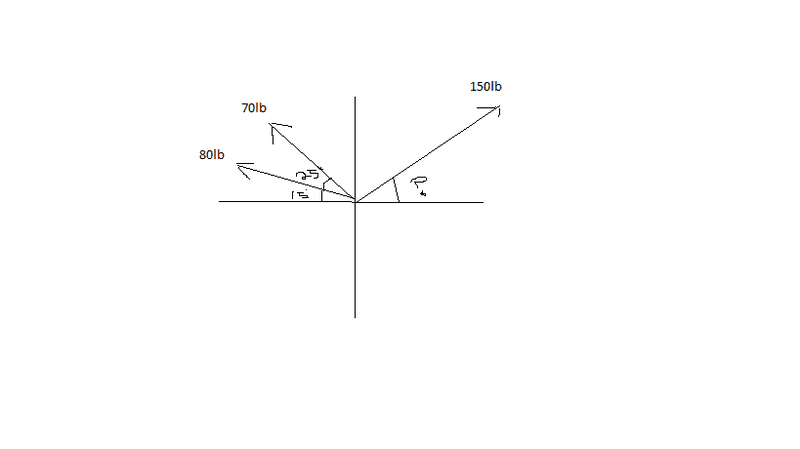# Adding Vectors:

## Homework Statement

10.0km [N] + 5.0km [E15S]

Cosine Law

## The Attempt at a Solution

If you draw it out you see that 10km is 90 degrees north. From the 10km end, you do E15S for 5km.

Therefore, a = 10km, b = 5km, c = ?, C = 90 - 15 = 75.

Therefore:

c^2 = 10^2 + 5^2 - 2(10)(5) Cos 75
c^2 = 99.12
c = 9.9

Sin Law to find the angle of the resultant

Sin A = Sin B
----- ------
a = b

Sin 75 Sin B
------- = -------
9.9 5

B = 29

So final answer is 9.9km [N29E]

Somehow the answer is 4.2[N35E]...

PeterO
Homework Helper

## Homework Statement

10.0km [N] + 5.0km [E15S]

Cosine Law

## The Attempt at a Solution

If you draw it out you see that 10km is 90 degrees north. From the 10km end, you do E15S for 5km.

Therefore, a = 10km, b = 5km, c = ?, C = 90 - 15 = 75.

Therefore:

c^2 = 10^2 + 5^2 - 2(10)(5) Cos 75
c^2 = 99.12
c = 9.9

Sin Law to find the angle of the resultant

Sin A = Sin B
----- ------
a = b

Sin 75 Sin B
------- = -------
9.9 5

B = 29

So final answer is 9.9km [N29E]

Somehow the answer is 4.2[N35E]...

Your answer is the correct answer to the problem you presented.

Either you miss read [and posted] the question, or you have compared your answer to the answer of a different question.

Even if you went 10km North then 5km due South you would still be 5 km from you starting point. There is no way you finish within 4.2 km of your starting point!!

Your answer is the correct answer to the problem you presented.

Either you miss read [and posted] the question, or you have compared your answer to the answer of a different question.

Even if you went 10km North then 5km due South you would still be 5 km from you starting point. There is no way you finish within 4.2 km of your starting point!!

Thanks. I also was confused how the displacement would be less than 5km.

Appreciate it for clarifying!

How would you do this one then?

10km [N25E] + 5km [E]?

SammyS
Staff Emeritus
Science Advisor
Homework Helper
Gold Member
One vector is due north. The other is 15° South of due East. The angle made by the direction of the vectors is 105°.

cos(105°) = -cos(75°)

PeterO
Homework Helper
One vector is due north. The other is 15° South of due East. The angle made by the direction of the vectors is 105°.

cos(105°) = -cos(75°)

When you add vectors you connect them head to to tail - that is why the angle is indeed 75° NOT 105°.

Sure the vectors separately will make an angle of 105°, if they have a common start point, but that is not how you add vectors.

PeterO
Homework Helper
Thanks. I also was confused how the displacement would be less than 5km.

Appreciate it for clarifying!

How would you do this one then?

10km [N25E] + 5km [E]?

Do i the same way you did the previous question. You will be working with an angle bigger than 90° this time.

PeterO
Homework Helper
Thanks. I also was confused how the displacement would be less than 5km.

Appreciate it for clarifying!

How would you do this one then?

10km [N25E] + 5km [E]?

You can use components of each vector if you wish.

10km [N25E] has a North component of about 9, and an East component about 4.2

When you add components your final vector will have components about 9 North and 9,2 East.

The final vector will be about 12.8 [N46E]

All those figures I approximated - I even guessed 46 for the angle, knowing that of the components had been 9 N and 9 East the angle would have been 45, so I just went a little bit bigger.
To get accurate components you use trig. and Pythagoras.

How the hell do you find this angle? see attached pic

says: determine the angle for which the resultant of the three forces is vertical.

#### Attachments

•Untitled.png
5.7 KB · Views: 491
PeterO
Homework Helper
How the hell do you find this angle? see attached pic

says: determine the angle for which the resultant of the three forces is vertical.

When you read the question did you realise that the statement in read means: "there is zero horizontal component to the resultant of the three forces?"

SammyS
Staff Emeritus
Science Advisor
Homework Helper
Gold Member
How the hell do you find this angle? see attached pic

says: determine the angle for which the resultant of the three forces is vertical.Hello SUCRALOSE . Welcome to PF !

You really should have posted this question in a new thread, rather than "hijacking" an existing link.

was not my intention to take over this thread, I start a new one. and I still do not get it.

PeterO
Homework Helper
was not my intention to take over this thread, I start a new one. and I still do not get it.

Can you answer my earlier question about what you saw in the wording please.